# Signed Integer Representations

Sign magnitude: the most significant bit is assigned to the algebraic sign.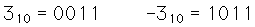Offset binary: subtract half the largest possible number to get the value represented. I.e., you use half the largest number as the "zero" of the scale. For four bits: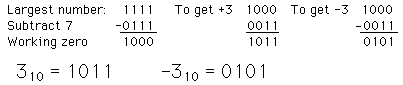2's Complement: negative integer is the complement of the positive integer plus one.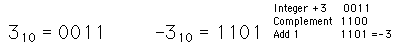# Other Integer Representations

Binary Coded Decimal (BCD or 8421 BCD): each individual digit of the decimal number is represented by a 4-bit binary number.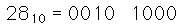Excess-3: Add 3 to the number, then represent by 4-bit binary number.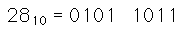4221 Code: Four bits represent 4,2,2,1 instead of 8,4,2,1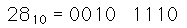Gray Code: Starting at zero, make one change in the least significant possible bit to take you to the next state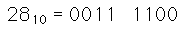Number Systems
Index

Electronics concepts

Digital Circuits

 HyperPhysics*****Electricity and magnetism R Nave
Go Back

# "Sign Magnitude" Representation

The most significant bit of a binary number can be used to represent the sign of the number, using the other bits to represent its magnitude. It is a natural kind of representation of signed integers, but is not used much because it is awkward for doing arithmetic compared to a system like 2's complement. There are also two zeros, since + and - zero will have a different code.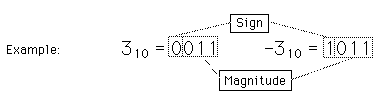Number Systems
Index

Electronics concepts

Digital Circuits

 HyperPhysics*****Electricity and magnetism R Nave
Go Back

# Offset Binary Representation

One logical way to represent signed integers is to have enough range in binary numbers so that the zero can be offset to the middle of the range of positive binary numbers. Then the magnitude of a negative binary number can be simply subtracted from that zero point. This system has the advantage of a simple binary progression from negative to positive numbers. It is useful for binary counters and for A/D and D/A conversion. It is awkward for computation compared to the 2's complement representation.

As a simple example, consider a 4-bit binary number with maximum number 15. Subtracting 7 gives the following representation: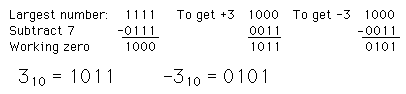Number Systems
Index

Electronics concepts

Digital Circuits

 HyperPhysics*****Electricity and magnetism R Nave
Go Back

# 2's Complement Representation

This code is the most widely used code for integer computation. Positive numbers are represented by unsigned binary numbers. Negative numbers are formed by the following procedure.

To produce the number -5:

 1. Write the binary number for 5 0101 2. Take the complement of it 1010 3. Add 1 1011

This code has the advantage that arithmetic operations are straightforward. Subtraction is accomplished by binary addition of the 2's complement. Multiplication also can be carried out.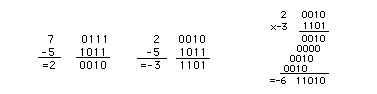Number Systems
Index

Electronics concepts

Digital Circuits

 HyperPhysics*****Electricity and magnetism R Nave
Go Back